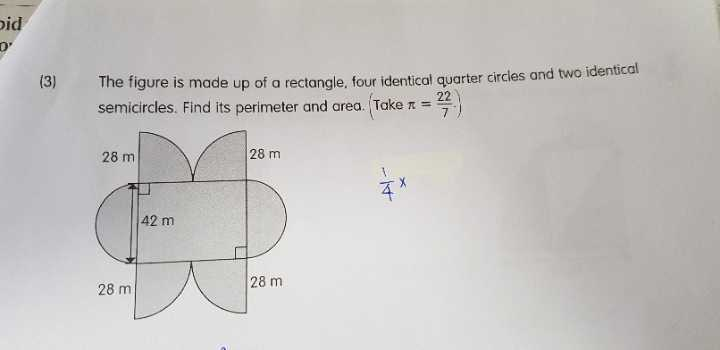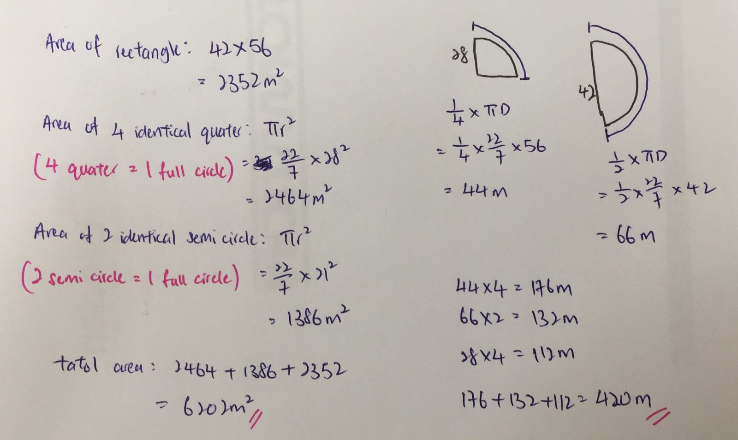# QuestionAnyonecan help?

This is a Geometry question.

 Big Circle Small Circle Line Rectangle Total Radius 28 21 Perimeter 176 132 112 420 Area 2464 1386 2352 6202

a) Perimeter

2 x 28 x 22/7 = 176

2 x 21 x 22/7 = 132

28 x 4 = 112

176 + 132 + 112 = 420cm

b) Area

22/7 x 28 x 28 = 2464

22/7 x 21 x 21 = 1386

42 x 56 = 2352

2464 + 1386 + 2352 =6202 cm^2

Why did u take 42×56?

length of the rectangle = 28 + 28 = 56

area of the rectangle = 42 x 56 = 2352

Thus, my solution is a s follows :

Its perimeter = (22/7 x 42) + [(22/7) x (28 + 28)] + (28 x 4) = 420

Its area = [(22/7) x 21 x 21] + [(22/7) x 28 x 28] + [42 x (28 + 28)] = 6202

Ans : Its perimeter = 420 cm; its area = 6202 sq cm.

0 Replies 0 Likes0 Replies 0 Likes

PERIMETER:

4 radius of quarter circles: 4 x 28 = 112m
circumference of all 4 quarter circles (4 quarter circle = 1 full circle): 22/7 x (28 x 2) = 176m
circumference of 2 semi-circles (2 semi circle = 1 full circle): 22/7 x 42 = 132m
Therefore, perimeter is 112 + 176 + 132 = 420m

AREA:

area of rectangle: 42 x (28 + 28) = 42 x 56 = 2352m²
(Note: The 28m comes from the radius of the quarter circles)
area of 4 quarter circles: 22/7 x 28 x 28 = 2464m²
area of 2 semi-circles: 22/7 x 21 x 21 = 1386m²
Therefore, area is 2352 + 2464 + 1386 = 6202m²

0 Replies 0 Likes